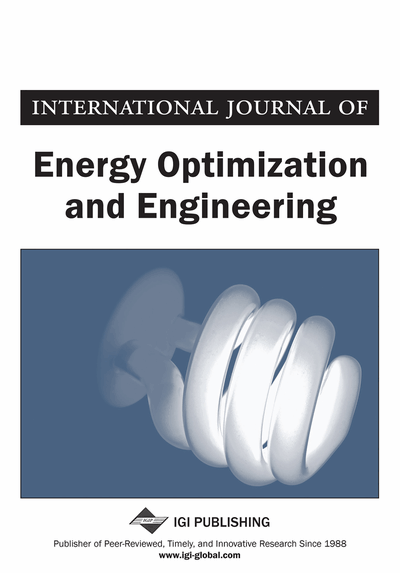# Gain Schedule PI Fuzzy Load Frequency Control for Two-Area Electric Power System: Load Frequency Control

Tawfiq H. Elmenfy (University of Benghazi, Libya)
DOI: 10.4018/IJEOE.2020070103
Available
\$29.50
No Current Special Offers

## Abstract

The use of proportional integral (PI) load frequency control (LFC) to ensure the stable and reliable operation of electric power system is practical important. Any imbalance between synchronous generators and consumption loads will cause frequency unstable within the complete power system. The purpose of the load frequency control (LFC) is to keep the power system frequency and the inter-area tie power as near equilibrium point. This article introduces a gain schedule PI fuzzy load frequency control (GLFC) applying to two area electric power system. The GLFC consists of two level control systems, where the PI controller in the conventional form and its parameters are tuned in real time by fuzzy system. A fuzzy rule base is constructed in the form set of IF-THEN that describe how to choose the PI parameters under different operating conditions. The simulation has been conducted in MATLAB Simulink package. The effectiveness of the GLFC is measured by comparison with conventional PI load frequency controller.
Article Preview
Top

## 1. Introduction

Frequency will be fixed in power system if there is a balance between generators and customer demand. The frequency of the power system is dependent on active power balance. As frequency is a common factor throughout the power system, a change in active power demand at one point is reflected throughout the power system by a change in frequency. Because there are many generators supplying power into the grid, these generators must be provided with LFC to control the generators output and maintain the frequency at a preset value (El-Sadek, 2004).

The gas turbines power plants had been used to improve the load frequency control in power system due to small time contsraint. The amount of these gas turbine plants must be distributed uniformly on the grid (WECC Tutorial, 2002).

In case of frequency drop at power plants the automatic LOAD-SHEEDING should initiate the first stage to frequency not lower that 49Hz with minimum 10 to 20% of the load and the load/frequency controller at power plants should try to recover the frequency balance between power generation and power demand by increase the megawatts of own generator to compensated the load demand.

However, if the load frequency control is unable to recover the frequency balancing, and the frequency of generators drops up to 47.5 Hz, the power stations automatically trip at this frequency without time delay. Running of power plants below this frequency are dangerous and will cause (danger vibrations and damage of the turbine blades).

In practical systems, the conventional PI controllers are used to control Load Frequency Control. To avoids the drawbacks of PI controllers, Numerous techniques have been introduced in the literatures. A fuzzy type controller is considered for LFC is introduced in Moitaba et al. (2011), the upper and lower bounds of membership functions are obtained by genetic algorithm. A genetic algorithm (GA) based fuzzy gain scheduling is proposed in Juang and Lu (2005), a fuzzy system is used to adaptively decide the PI controller gains, to reduce the number of fuzzy rule base, the fuzzy system designed by automatically by genetic algorithm. The interconnected two area systems are modeled and simulated be PI fuzzy controller with sliding gain for improved performance specification like settling time and overshoot is introduced in Prakash et al. (2009). Control methodology developed using conventional PI controller, Artificial Neural Network (ANN) and Fuzzy Logic controller (FLC) for three area interconnected power system is introduced in Prakash and Sinha (2001), a comparison of that controller shows the superiority of the proposed controller over conventional PI controller. Power system load frequency control by modified dynamic neural networks controller proposed in Sabahi et al. (2007), the controller has dynamic neurons in hidden layer and conventional neurons in other layers. For considering the sensitivity of power system model, the neural network emulator used to identify the model simultaneously with control process. new robust load frequency controller for two area interconnected power system is presented in Venkata and Jayaram (2008). The Genetic algorithm (GA) controller consists of two crisp inputs namely deviation of frequency and the other is derivative of frequency deviation. The output of the Genetic algorithm controller is the control input to each area. The paper in Tammam et al. (2012) studies control of load frequency in single and two area power systems with fuzzy like PID controller. The study, multi-objective genetic algorithm is used to determine the parameters of the fuzzy like PID controller according to the system dynamics, the controller has been compared with the conventional PID controllers tuned by Ziegler-Nicholas method and Particle Swarm Optimization technique. The overshoots and settling times with the proposed Genetic-PID controller are superior to the outputs of the same characteristics of the conventional PID controllers. Decentralized fuzzy logic load frequency controller (Ellithy & Elmetwally, 2012), consists of two internal fuzzy logic controllers namely, the PD and PI-like fuzzy controller.

## Complete Article List

Search this Journal:
Reset
Volume 12: 1 Issue (2023): Forthcoming, Available for Pre-Order
Volume 11: 4 Issues (2022): 2 Released, 2 Forthcoming
Volume 10: 4 Issues (2021)
Volume 9: 4 Issues (2020)
Volume 8: 4 Issues (2019)
Volume 7: 4 Issues (2018)
Volume 6: 4 Issues (2017)
Volume 5: 4 Issues (2016)
Volume 4: 4 Issues (2015)
Volume 3: 4 Issues (2014)
Volume 2: 4 Issues (2013)
Volume 1: 4 Issues (2012)
View Complete Journal Contents Listing